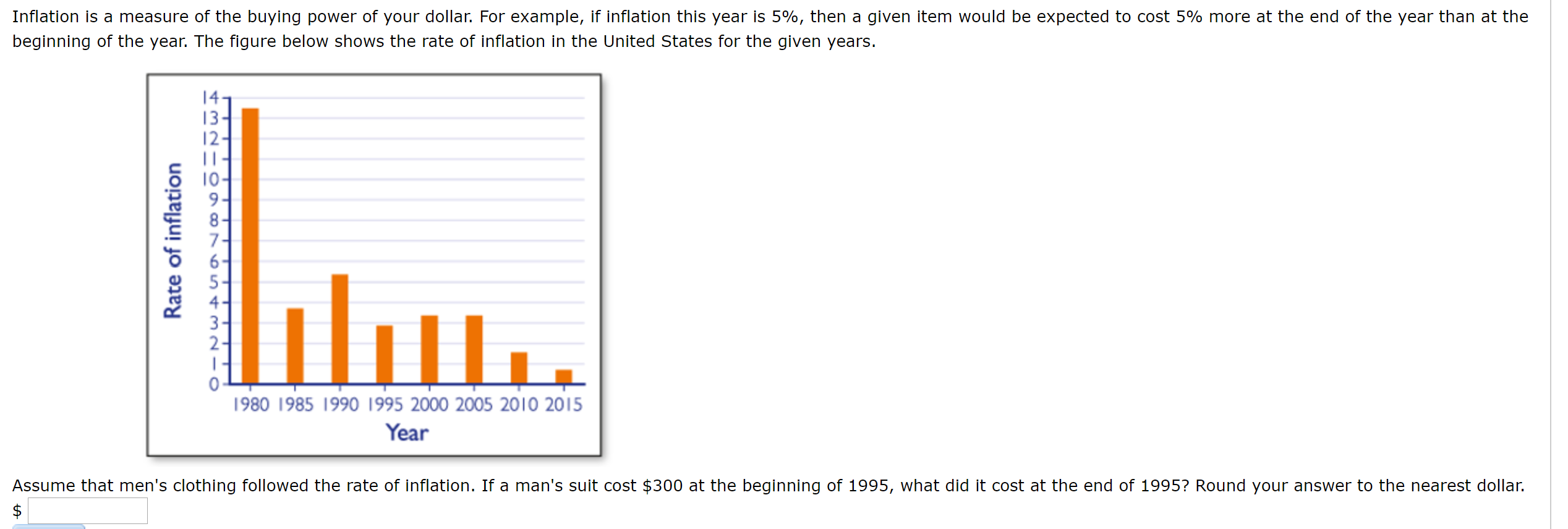# Inflation is a measure of the buying power of your dollar. For example, if inflation this year is 5%, then a given item would be expected to cost 5% more at the end of the year than at thebeginning of the year. The figure below shows the rate of inflation in the United States for the given years.1413-7.3-2-П1980 1985 1990 1995 2000 2005 2010 2015YearAssume that men's clothing followed the rate of inflation. If a man's suit cost \$300 at the beginning of 1995, what did it cost at the end of 1995? Round your answer to the nearest dollar.Rate of inflation

Question
27 views

Inflation is a measure of the buying power of your dollar. For example, if inflation this year is 5%, then a given item would be expected to cost 5% more at the end of the year than at the beginning of the year. The figure below shows the rate of inflation in the United States for the given years.

Assume that men's clothing followed the rate of inflation. If a man's suit cost \$300 at the beginning of 1995, what did it cost at the end of 1995? Round your answer to the nearest dollar.help_outlineImage TranscriptioncloseInflation is a measure of the buying power of your dollar. For example, if inflation this year is 5%, then a given item would be expected to cost 5% more at the end of the year than at the beginning of the year. The figure below shows the rate of inflation in the United States for the given years. 14 13- 7. 3- 2- П 1980 1985 1990 1995 2000 2005 2010 2015 Year Assume that men's clothing followed the rate of inflation. If a man's suit cost \$300 at the beginning of 1995, what did it cost at the end of 1995? Round your answer to the nearest dollar. Rate of inflation fullscreen
check_circle

Step 1

Since men’s clothing followed the rate of inflation. In 1995, the cost of man’s suit is \$300 at the beginning. Then the cost would be 5% more at the end of the year.

Step 2

Then,

Cost of the suit at the beginning of the year = \$300

5% more cost = 300 + .05*300

...

### Want to see the full answer?

See Solution

#### Want to see this answer and more?

Solutions are written by subject experts who are available 24/7. Questions are typically answered within 1 hour.*

See Solution
*Response times may vary by subject and question.
Tagged in

### Math